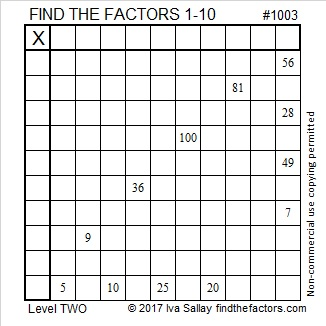# 1003 and Level 2

There is only one way to write the numbers 1 to 10 in both the first column and the top row so that you create a multiplication table and the clues in the puzzle belong where they are. Can you find that way? It easy difficult. Give it a try!Print the puzzles or type the solution in this excel file: 10-factors-1002-1011

1003 is the hypotenuse of a Pythagorean triple:
472∗885∗1003 which is (8-15-17) times 59

1003 is also palindrome 1101011 in BASE 3
because 3⁶ + 3⁵ + 0(3⁴) + 3³ + 0(3²) + 3¹ + 3⁰ = 1003

• 1003 is a composite number.
• Prime factorization: 1003 = 17 × 59
• The exponents in the prime factorization are 1 and 1. Adding one to each and multiplying we get (1 + 1)(1 + 1) = 2 × 2 = 4. Therefore 1003 has exactly 4 factors.
• Factors of 1003: 1, 17, 59, 1003
• Factor pairs: 1003 = 1 × 1003 or 17 × 59
• 1003 has no square factors that allow its square root to be simplified. √1003 ≈ 31.670175This site uses Akismet to reduce spam. Learn how your comment data is processed.